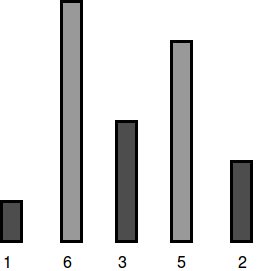# The Hurdle Race Hackerrank Problem Solution- Coder In Me

Dan is playing a video game in which his character competes in The Hurdle Race. Hurdles are of varying heights, and Dan has a maximum height he can jump. There is a magic potion he can take that will increase his maximum height by 1 unit for each dose. How many doses of the potion must he take to be able to jump all of the hurdles?

Given an array of hurdle heights h, and an initial maximum height Dan can jump, k, determine the minimum number of doses Dan must take to be able to clear all the hurdles in the race.

For example, if h=[1,2,3,3,2] and Dan can jump 1 unit high naturally, he must take 3-1=2 doses of potion to be able to jump all of the hurdles.

#### Input Format of The Hurdle Race

The first line contains two space-separated integers n and k, the number of hurdles and the maximum height Dan can jump naturally.
The second line contains space-separated integers h[i] where 0<=i<=n .

1<=n,k<=100

1<=h[i]<=100

#### Output Format

Print an integer denoting the minimum doses of magic potion Dan must drink to complete the hurdle race.

5 4
1 6 3 5 2

2

##### Explanation 0

Dan’s character can jump a maximum of units, but the tallest hurdle has a height of :To be able to jump all the hurdles, Dan must drink doses.

5 7
2 5 4 5 2

0

##### Explanation 1

Dan’s character can jump a maximum of units, which is enough to cross all the hurdles:Because he can already jump all the hurdles, Dan needs to drink doses.

HOW TO THINK:

The solution of the Hurdle Race

We know that Dan can jump any hurdle that has height h[i]<=k. To find the minimum number of beverages he must drink to clear all the hurdles, we simply need to determine the difference between the height he can alreadyjump and the height he needs to jump in order to complete the race. Note that if each h[i]<=k, he can already clear all the hurdles and the answer is 0.

## Solution

1. Find the maximum height of any hurdle, hmax.
2. Calculate the difference between hmax and k as diff=hmax-k
3. If diff>0, then the difference corresponds to the number of beverages he must drink and we print the value of diff as our answer.
• If diff<=0, then he is already capable of clearing all the hurdles and we print 0 as our answer.

THINK

Dan’s character can jump up to a maximum height of k, and the hurdles are of varying heights. So if his character drinks magic potion his maximum height increases by 1 unit.

Thus, if we can get the maximum height of the hurdle and given the maximum height his character can jump we can find the minimum number of potions his character needs to easily jump all the hurdles.

HOW TO CODE:

We get an array height which contains the heights of the hurdles. We find the maximum height of the hurdles from the array using a loop. After doing so we find out the no of potions required by simply subtracting k maximum height, and we return the result so obtained.

THE CODE:

C+ solution

#include <iostream>

using namespace std;

int main()
{
int n, k, hurdle, max_hurdle = 1;
cin >> n >> k;
while(cin >> hurdle)
if(hurdle > max_hurdle) max_hurdle = hurdle;

cout << (max_hurdle - k >= 0 ? max_hurdle - k : 0) << endl;

return 0;
}

#include <bits/stdc++.h>

using namespace std;

int hurdleRace(int k, vector <int> height) {

// Complete this function

int maxh=height;

for(int i=1;i<height.size();i++)

{

if(height[i]>maxh)

{

maxh=height[i];

}

}

if(maxh>k)

return maxh-k;

else

return 0;

}

int main() {

int n;

int k;

cin >> n >> k;

vector<int> height(n);

for(int height_i = 0; height_i < n; height_i++){

cin >> height[height_i];

}

int result = hurdleRace(k, height);

cout << result << endl;

return 0;

}
##### JAVA
import java.io.*;
import java.util.*;
import java.text.*;
import java.math.*;
import java.util.regex.*;

public class Solution {

public static void main(String[] args) {
Scanner in = new Scanner(System.in);
int n = in.nextInt();
int k = in.nextInt();
int[] height = new int[n];
for(int height_i=0; height_i < n; height_i++){
height[height_i] = in.nextInt();
}
Arrays.sort(height);
System.out.println(Math.max(0, height[n-1]-k));
}
}
##### PYTHON
#!/bin/python

import sys

n,k = raw_input().strip().split(' ')
n,k = [int(n),int(k)]
height = map(int, raw_input().strip().split(' '))
print max(0,m-k)